Project

# Embedded PID Temperature Control, Part 6: Ziegler–Nichols Tuning

March 07, 2016 by Robert Keim

## In this article, we will use a simple, proven tuning procedure to find effective values for proportional, integral, and derivative gain.

In this article we will use a simple, proven tuning procedure to find effective values for proportional, integral, and derivative gain.

### Previous Articles in This Series

Before we get started, here is the PID control system diagram presented previously: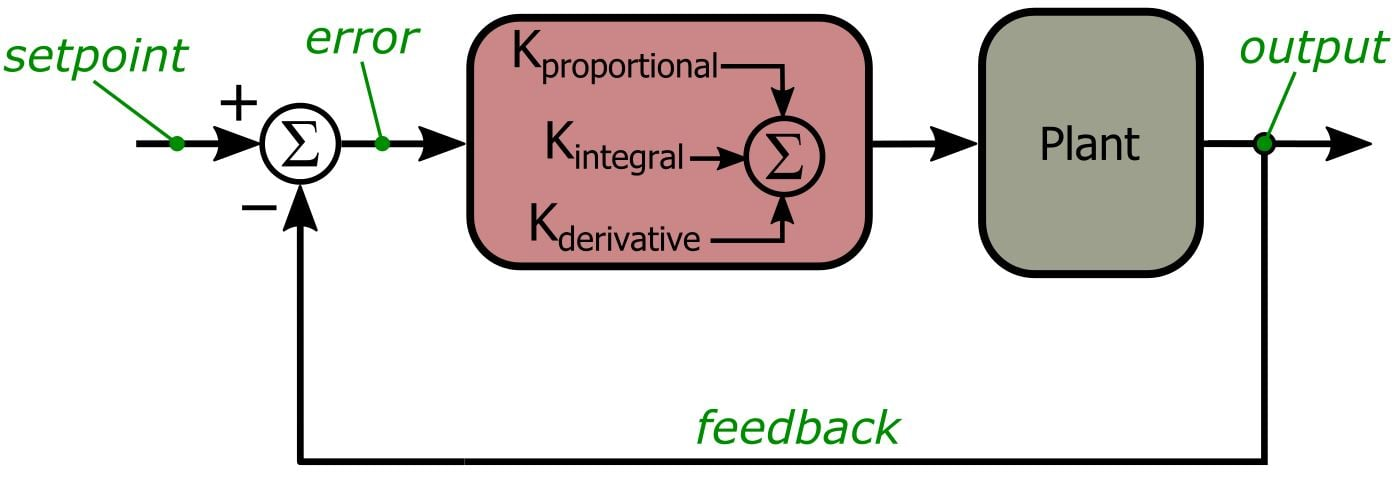And here are PID-related portions of the schematic: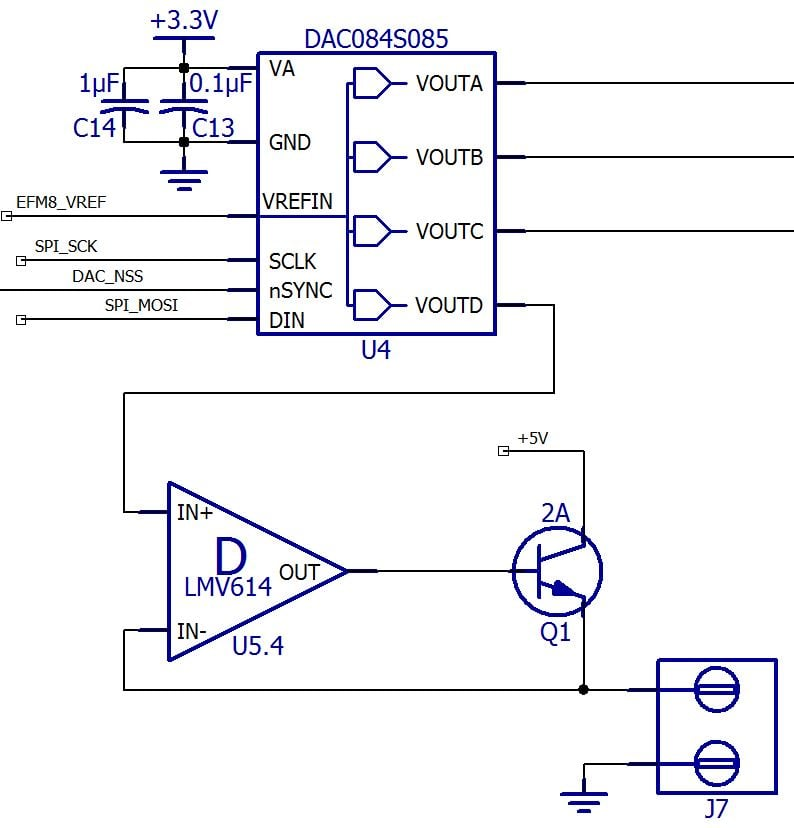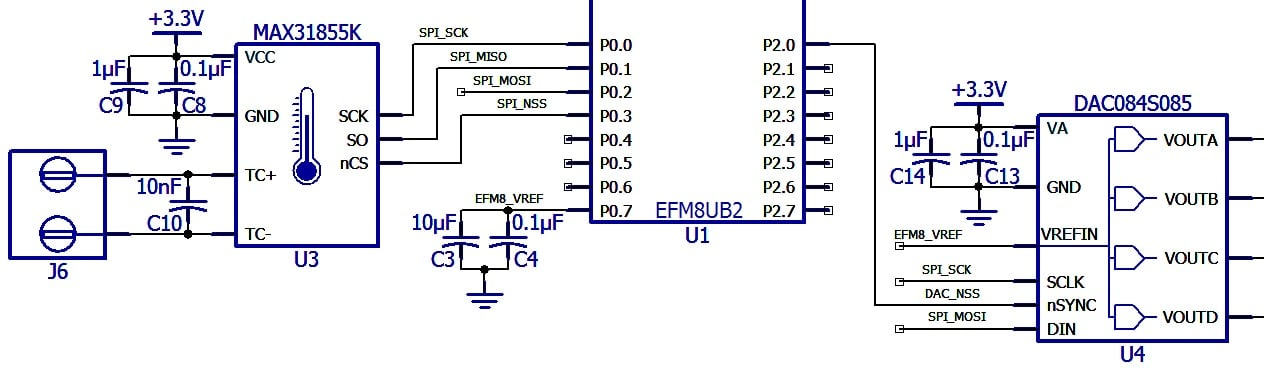### The Limitations of Guess-and-Check

In the previous article we employed some basic system analysis followed by crude experimentation to determine workable PID gain parameters. The resulting performance was quite good—but then again, we only tested the system with one specific control task, so we don’t know much about what the controller might do under different operating conditions. We also don’t know how much better the performance could be. It’s all well and good to be satisfied when you see adequate performance, but what if the system could be significantly improved simply by changing the gain values? The guess-and-check approach is also rather impractical, or at least tiresome. The process was time-consuming even with this little experimental temperature controller—what would you do if each control run took an hour instead of a few minutes? My point here is that efficient, effective PID tuning is an essential part of control-system design.

It should come as no surprise that the people designing jet fighters and Mars rovers need something way better than guess-and-check. Sophisticated PID software packages or simulation environments are probably the method of choice for most modern control systems; we, on the other hand, will use a simple, time-tested technique known as the Ziegler–Nichols method.

### Ziegler–Nichols

This procedure was first described in a paper published in 1942—credit to Ziegler and Nichols for coming up with a tuning method that has survived almost 75 years of overwhelming technological development. The process starts with a proportional-gain-only system. You increase the P gain until the system exhibits oscillations that are sustained (i.e., stable in terms of amplitude) and regular (i.e., stable in terms of period); the oscillation does not need to be centered around the setpoint. This is the only tiresome part of the Ziegler–Nichols method. The rest is just math.

Once you have sustained, regular oscillation, you record the proportional gain and measure the oscillation period. These values are referred to as the ultimate gain (KU) and the ultimate period (PU), respectively. Now you calculate KP, KI, and KD according to the following table:

 KP TI TD P-only control KU/2 PI control KU/2.2 PU/1.2 PID control KU/1.7 PU/2 PU/8

Note that the integral and derivative columns are labeled TI and TD instead of KI and KD. These “T” variables refer to the time constant used when calculating the integral or derivative. For our discrete-time controller, we use the following relationships to determine KI and KD:

$K_I=K_P\left(\frac{T}{T_I}\right)\ \ \ \ \ \ \ \ \ K_D=K_P\left(\frac{T_D}{T}\right)$

where T is the PID interval, i.e., the amount of time between successive executions of the routine that calculates the PID output and updates the control circuitry. The current version of our temperature controller uses a PID interval of 2 seconds.

### Seeking Oscillation

So first we need to find the ultimate gain and period. Feedback systems often burden engineers with the problem of preventing oscillation; there is something relaxing about encouraging oscillation and even feeling a sense of satisfaction when it occurs with sufficient regularity.

In the previous article we saw that a P-only system with KP = 70 does not produce stable oscillation: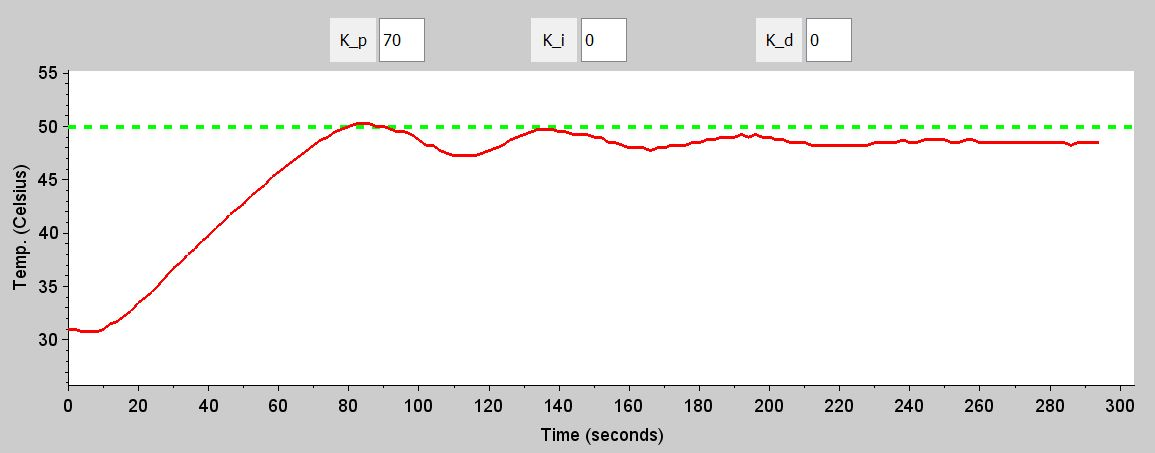So let’s increase KP to 90 and see what happens.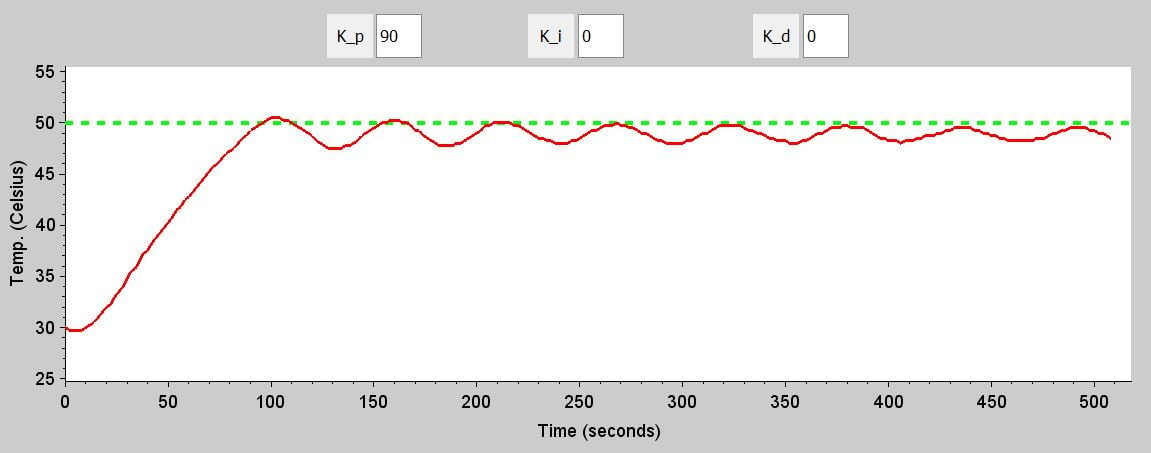This is looking better, but the amplitude of the oscillations clearly decreases over time. Let’s try KP = 100: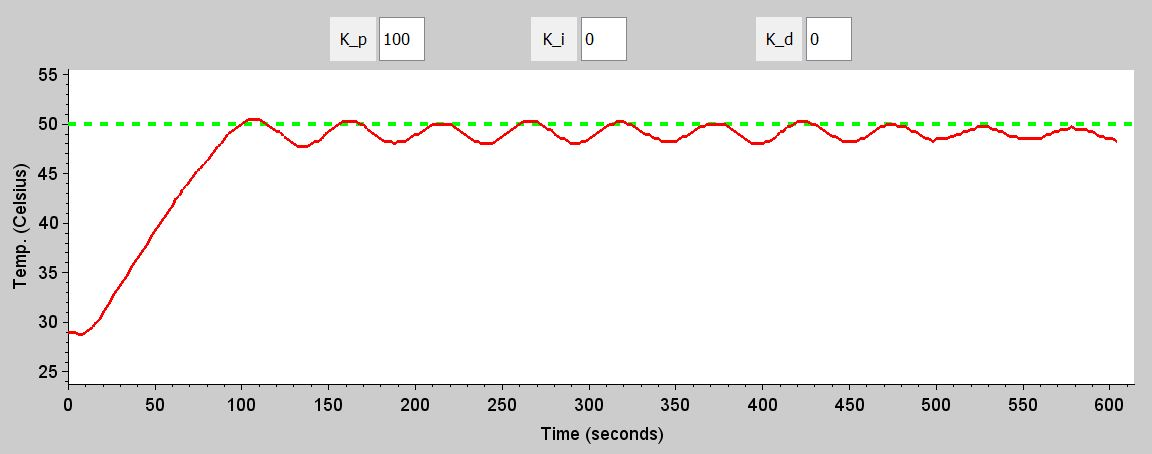I would consider this borderline. The oscillations are regular but not quite sustained (the decreasing amplitude is not really apparent until the last three cycles). Here’s KP = 110: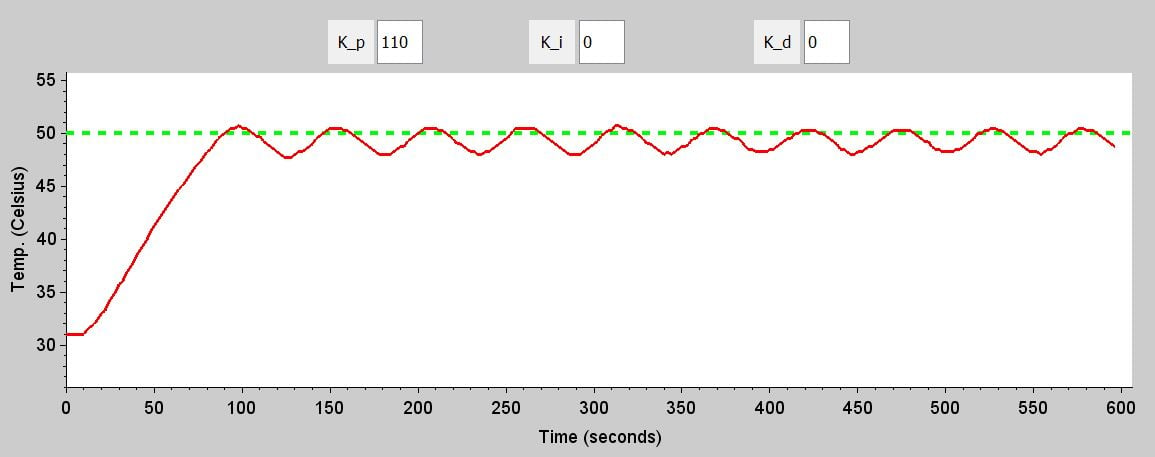This looks good to me. I don’t notice any significant irregularity or reduction in amplitude. So we will say that the ultimate gain is 110.

$K_P\ for\ sustained,\ regular\ oscillation\ is\ 110\ \ \Rightarrow\ \ K_U=110$

As indicated in the following plot, the ultimate period is about 55 seconds.

$T_U=55\ seconds$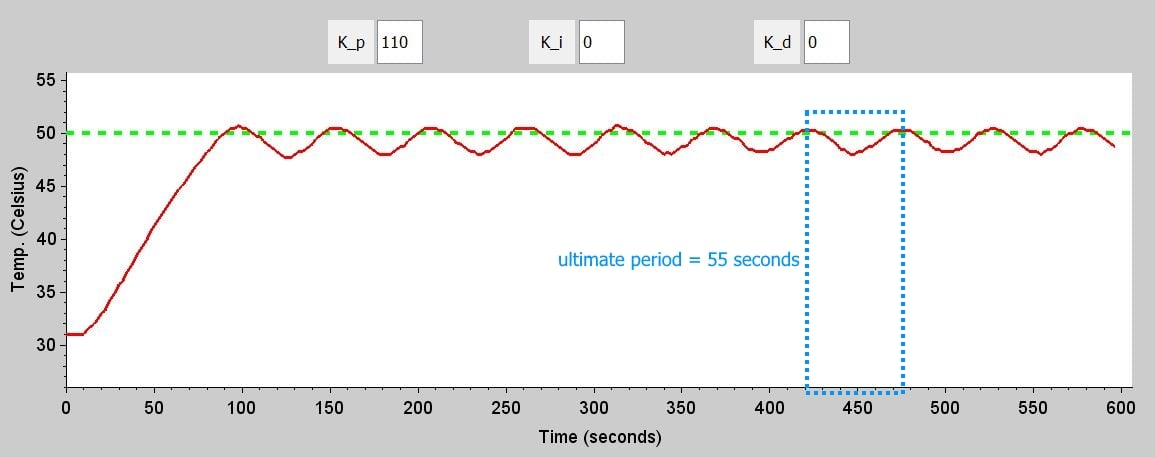### Calculations

The first table shows the Ziegler–Nichols values for the proportional gain, integral time constant, and derivative time constant assuming KU = 110 and TU = 55 seconds. The second table has gain values instead of time constants for the integral and derivative terms, assuming that the PID interval is 2 seconds. All values in the second table are rounded to the nearest integer; these are the values that we will actually use in the system, which does not support non-integer gains.

 KP TI TD P-only control 55 PI control 50 45.8 PID control 64.7 27.5 6.9

 KP KI KD P-only control 55 PI control 50 2 PID control 65 5 223

### Results

We will compare the performance of different system configurations based on convergence time, which we will define as follows: the interval between the time at which the measured temperature first reaches the setpoint and the time at which the measured temperature remains directly on top of or immediately adjacent to the setpoint line. (This convergence criterion corresponds to a range of about 1°C above or below the setpoint temperature.) We want the interval to begin when the measured temperature first reaches the setpoint (rather than at the beginning of the control run) because this should minimize errors associated with variations in the initial system state (the dominant concern being variations in the measured temperature at the beginning of the control run).

First let’s look at the Ziegler–Nichols P-only system: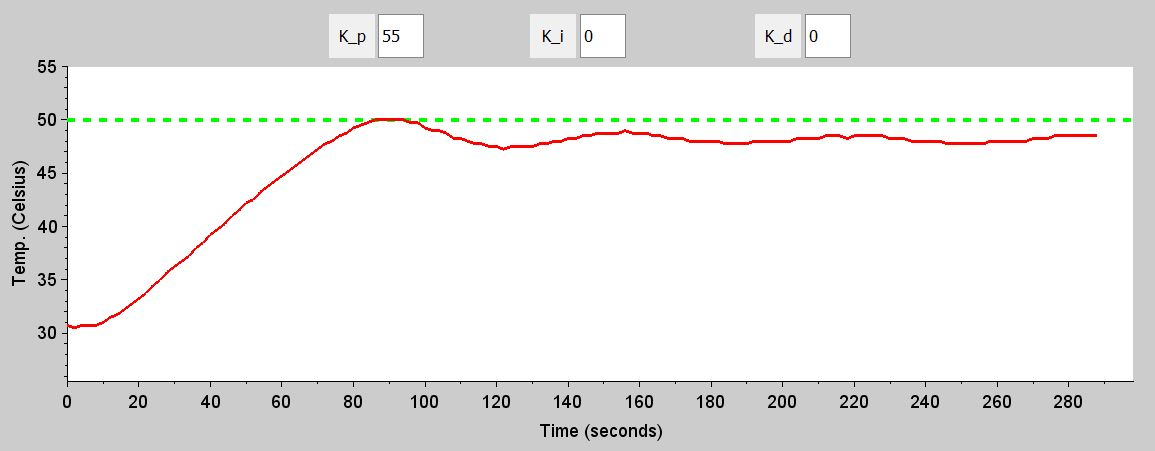Unsurprisingly, this system doesn’t even converge. There’s only so much you can do with a P-only system.

Here is the Ziegler–Nichols PI system: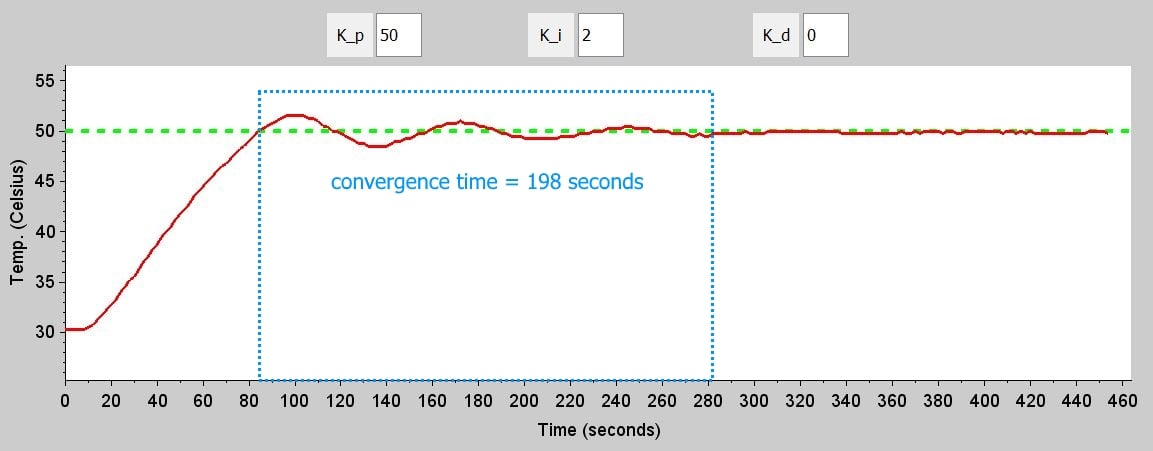This is good—probably about the best we can do without any derivative gain. Actually, after all the time I have spent experimenting with this system, I cannot remember a better PI control run. As marked in the plot, the convergence time was 198 seconds. Note also the reasonable amount of overshoot. It’s important to keep in mind that the Ziegler–Nichols method includes a tuning procedure specifically for PI control because in many situations it is acceptable or even preferable to use only proportional and integral gain. Derivative action can be problematic when the system is susceptible to major disturbances or noise in the measured variable.

Now we’re ready for the full PID: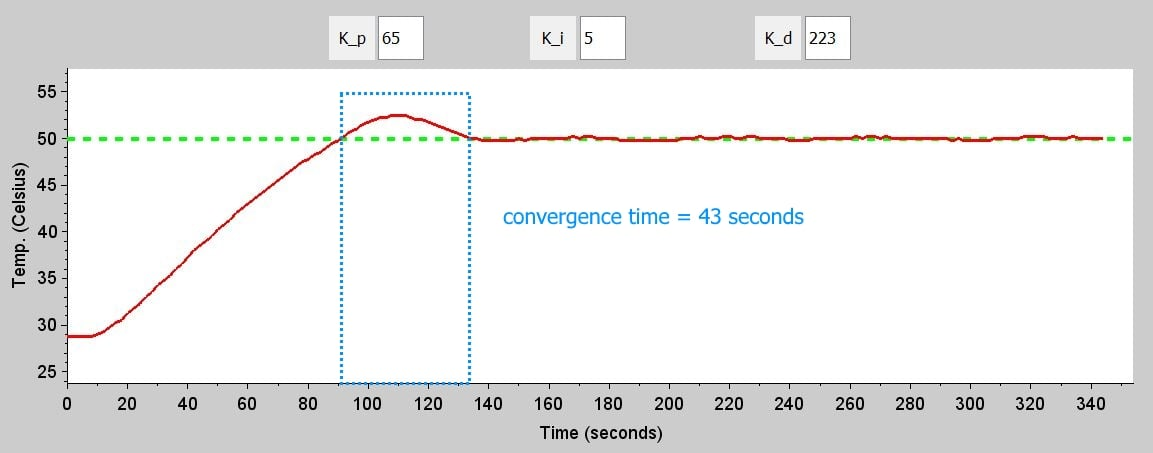Nicely done, Ziegler and Nichols. This far surpasses any control run I’ve seen so far. The convergence time has decreased from 198 seconds to 43 seconds, and the overshoot has increased by only about 1°C. Despite what I said above about the dangers of derivative gain, it is clearly beneficial in this system. I suppose this is not too surprising—we have no major disturbances, and there is not much noise in the measured temperature (in part because the temperature measurements are effectively filtered by the limited resolution of the MAX31855 and the relatively long PID interval).

One weakness with the standard Ziegler–Nichols approach is the overshoot. In our system, and many other systems, this amount of overshoot is no problem. But there are situations in which overshoot is unacceptable—an extreme example is a substance that will ignite if the temperature goes too far above the setpoint. So before we finish up, we’ll look at tuning parameters that are intended to minimize overshoot. This article from Microstar Laboratories includes a table with the parameters for this “no overshoot” tuning method, as well as for two additional methods that differ from the standard Ziegler–Nichols technique.

 KP TI TD No overshoot KU/5 TU/2 TU/3

The corresponding rounded gains are KP = 22, KI = 2, and KD = 202. Here are the results: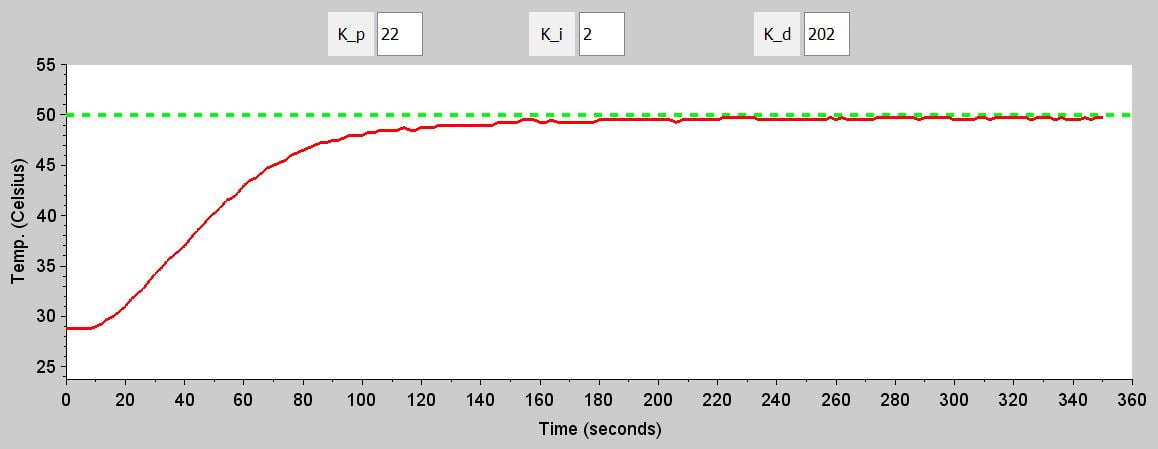The method succeeded in eliminating overshoot, to the serious detriment of convergence time. We can’t directly compare this run to previous runs because our convergence-time rule doesn’t work when the measured temperature never crosses the setpoint, and because this run never quite converges according to our previous criterion. But we can get a general idea from the overall time to near-convergence. With the Ziegler–Nichols PID system, the overall time to convergence was 134 seconds, whereas the “no overshoot” PID system comes very close to convergence at 275 seconds.

### Conclusion

We now understand the value of proven tuning methods. We had great success with the Ziegler–Nichols approach, but other methods are available. With this particular temperature controller, the Ziegler–Nichols procedure achieved performance that looks close to optimal. However, it’s important to understand that there is nothing magical about Ziegler–Nichols or any other method. If the system’s performance is not adequate, then the gain values generated by the method become a starting point for further observation and refinement.